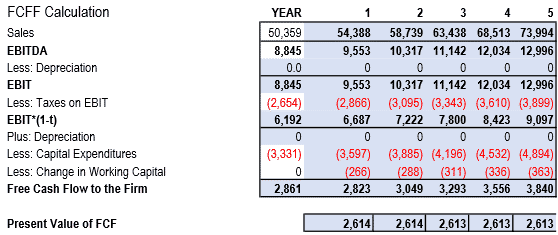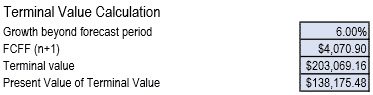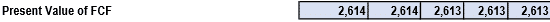# Explaining the DCF Valuation Model with a Simple Example

Discounted Cash Flow (DCF) valuation is one of the fundamental models in value investing. The model is used to calculate the present value of a firm by discounting the expected returns to their present value by using the weighted average cost of capital (WACC).

How to perform a DCF valuation

There are certain steps in performing a DCF valuation. These are:

1. Calculate the WACC

The weighted average cost of capital is fundamental to the capital asset pricing model (CAPM). The WACC is calculated as the weighted average of a firm’s cost of debt and cost of equity.

Assuming that you have invested in Novartis (NYSE: NVS), the steps for the calculation of WACC are the following:a) The first step is to find the cost of debt and the cost of equity.

The cost of debt is derived as follows:

The company’s total debt is \$16.33 billion and the interest expenses are \$655.0 million. The interest expenses will save Novartis \$655 x 30% (tax rate) = \$196.50 million. So, the after-tax cost of debt Rd = (\$655.0 – \$196.5) / \$16.33 = 2.81%

The cost of equity is calculated using the formula Rs = RRF + (RPM * b), where,

• RRF: the risk-free rate
• RPM: the return that the market expects
• b: the stock’s beta (systemic risk)

Therefore, for a risk-free rate of 5.00%, an expected market return of 5.10% and a b=0.75, the cost of equity is:

Rs = RRF + (RPM * b) = 5.00% + (5.10% x 0.75) = 8.83%

b) The second step is to calculate the weights of debt and equity

First, you need to find the Market value added (MVA) of the company, which represents the difference between the current market value of a firm and its book value. Novartis is currently trading at \$75.21 and the number of shares outstanding is 2.37 billion. Therefore, the company’s market value is \$75.21 x 2.37 = \$178.25 billion. Given the company’s book value per share (BVPS) of \$31.57, its book value is \$31.57 x 2.37 = \$74.82 billion. Therefore, the market value added is market value – book value = \$178.25 – \$74.82 = \$103.43 billion.

Now that you know the MVA and the total debt, you added them to derive the weights of debt and equity. Therefore:

The book value of debt + MVA of equity = \$16.33 + \$103.43 = \$119.76. Therefore, the weight of debt Wd = \$16.33 / \$119.76 = 13.63% and the weight of equity We = \$103.43 / \$119.76 = 86.37%.

c) The final step is to calculate the WACC

The formula for WACC is (Rd*Wd) + (Rs*We). Therefore:

WACC = (2.81% x 13.63%) + (8.83% x 86.37%) = 0.38% + 7.62% = 8.00%.

1. Discounting by the WACC

The goal of a DCF valuation is to derive the fair value of the stock and determine whether it trades above this value (overvalued) or below this valued (undervalued). Remember that value investing is set out to find undervalued stocks, i.e. stocks that trade below their fair value and, therefore, they have a room for growth.

a) Once you know the WACC you can create a spreadsheet for the calculation of the free cash flow to the firm (FCFF) by using the sales and EBITDA of the current year, and assuming a sales growth of 8% and a tax rate of 30% for the next 5 years. Then, you discount the FCFF to its present value, using the WACC. The changes in working capital are discounted using the WC/Sales ratio (working capital over sales), which in this case is \$3,331 / \$50,359 = 6.61%.Here is an example of the calculations:

Sales: 50,359 x (1+8%) = \$54,388 x (1+8%) = \$58,739 x (1+8%) = \$63,438 x (1+8%) =\$68,513 x (1+8%) = \$73,994

EBITDA: \$8,845 x (1+8%) = \$9,553 x (1+8%) = \$10,317 x (1+8%) = \$11,142 x (1+8%) = \$12,034 x (1+8%) = \$12,996

Since there are depreciation expenses, EBITDA is equal to EBIT.

Taxes on EBIT: \$8,845 x 30% and so on up to year 5.

Capex: 3,331 x (1+8%) = \$3,597 x (1+8%) = \$3,885 x (1+8%) = \$4,196 x (1+8%) = \$4,532 x (1+8%) = \$4,894.

Changes in WC: 6.61%* (\$54,388-\$50,359) = 266 and so on up to year 5.

FCFF: EBIT (1-t) – Capex – Changes in WC.

PV of FCFF: FCFF / (1+WACC)1 for year 1, ^2, for year 2 and so on up to year 5.

b) Then, you need to calculate the terminal value, assuming a growth of 6% beyond the forecast period. Therefore, the FCFF in the year 5 with a growth of 6% = \$3,840 x (1+6%) = \$4,070.90, and the terminal value is \$4,070.90 / (WACC – growth) = \$4,070.90 / (8% – 6%) = \$4,070.90 / 2% = \$203,544.90. Then, you discount the terminal value to its present value, using the WACC. So, the Present Value of Terminal Value is \$203,544.90 / (1+8%)5 = \$138,175.48.Calculating the total PV of the FCF = \$13,067.1c) Then, you need to calculate the equity value of the firm by:1. Add the PV of the terminal value to find the total equity value. So, \$13,067.1 + 138,175.48 = \$151,242.6
2. Deduct the debt of last year (in this case of 31/12/2015) to find the net asset value. So, \$151,242.6 – \$21,901 = \$129,341.6.d) Finally, you need to adjust the net asset value for the present day, as follows:So, according to the DCF valuation, Novartis is overvalued, as it trades at \$75.21 and the DCF shows that the value per share should be \$59.49 based on the company’s FCFF.

Some limitations of the DCF valuation

DCF Valuation is an extremely useful tool, provided that the growth rate assumed for the series of period is justifiable by the firm’s operating performance. Although the growth rate cannot be accurately estimated, a firm that is expanding and seek to enter into new markets is more likely to sustain an average growth of 10% for the next five years than a company that is contracting or losing market share. Also, the WACC calculation may not really apply to the real world.

Another area that requires attention is the capex projections. In the above example, we assumed Novartis’ capex is growing at 8% annually over the next five years. In the real world, this may not be so true. If, for example, a company is expanding in year 3, its capex will be higher in year 3 and lower in years 1,2,4 and 5. So, before using a constant growth for Capex, you need to dig into the company’ balance sheet to investigate the trend of capex throughout the investigation period.